# Bubble Sort in C++ Programming

###### Sorting in C++

In this lesson, we will understand what is Bubble Sort in C++ Programming along with some examples.

## What is Bubble Sort in C++?

A Bubble Sort is a sorting technique used in C++ to sort elements of an array in ascending or descending order.

In this sorting technique, elements are sorted in ascending order by repeatedly swapping the larger element with the smaller element. While in descending order, sorting is performed by repeatedly swapping the smaller element with the larger element.

To clearly understand the bubble sorting technique, let's go through its algorithm step by step with an example.## Bubble Sort Visualization

Suppose we have seven numbers stored in an array as shown below.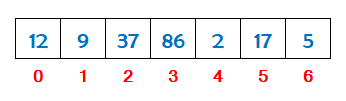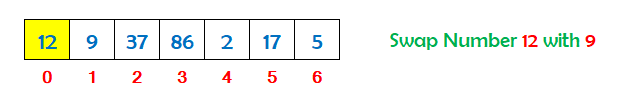Take the 1st number 12 and compare it with the 2nd number 9. 12 is greater than 9 so swap both the numbers.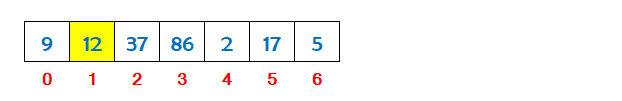Now take the 2nd number 12 and compare it with the 3rd number 37. 12 is not greater than 37 so leave it.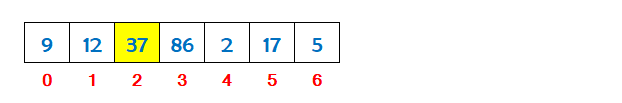Now take the 3rd number 37 and compare it with the 4th number 86. 37 is not greater than 86 so leave it.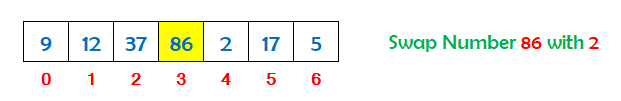Now take the 4th number 86 and compare it with the 5th number 2. 86 is greater than 2 so swap both the numbers.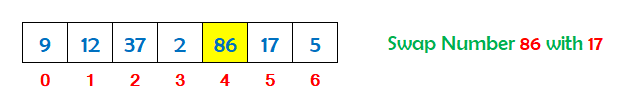Now take the 5th number 86 and compare it with the 6th number 17. 86 is greater than 17 so swap both the numbers.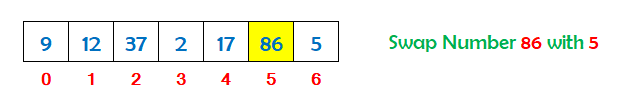Now take the 6th number 86 and compare it with the 7th number 5. 86 is greater than 5 so swap both the numbers.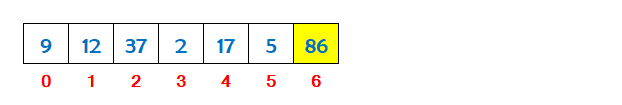So we can see that the largest number has shifted towards the end of the array. To arrange the other numbers also, we have to repeat the above process five times more from index 0 to index 4.

If there are ten number in an array, we have to repeat the above process nine times to sort the entire array.

## Algorithm for Bubble Sort

An algorithm is the steps used to implement the bubble sort in a c++ program.

Assume we have N numbers stored in an array. To implement the bubble sort on N numbers, the steps are as follows.

• Define an array to store N numbers for bubble sort. Suppose we have defined an array with the name num.
• Run an outer loop i from 0 to N-1 to repeat the process of bubble sort.
• Run an inner loop j inside the body of the outer loop i for bubble sort from 0 to N-1-i.
• Inside the body of the inner loop j, check if the value at num[j] is greater than the value at num[j+1].
• If the value at num[j] is greater than value at num[j+1] then swap the numbers.
• Now print the sorted array on the screen outside the body of the outer loop i.

## C++ Program for Bubble Sort

A C++ program to sort an array of numbers in ascending order using the bubble sort technique.

#### Bubble Sort (List of Numbers in Ascending Order)

``````#include <iostream>
#include <conio.h>

using namespace std;

int main()
{
// define an array
int num[]= {12,9,37,86,2,17,5};
int i,j,t;

cout<<"Array before Bubble Sort"<<endl;
for(i=0; i<7; i++)
{
cout<<num[i]<<" ";
}

// run an outer loop i from 0 to N-1 to repeat the process of bubble sort
for(i=0; i<6; i++)
{
// run an inner loop j for bubble sort from 0 to N-1-i
for(j=0; j<6-i; j++)
{
// now check if the value at num[j] is greater than value at num[j+1]
if(num[j]>num[j+1])
{
// if the value is greater then swap the numbers
t=num[j];
num[j]=num[j+1];
num[j+1]=t;
}
}
}

// print the sorted list
cout<<"\n\nArray after Bubble Sort\n";
for(i=0; i<7; i++)
{
cout<<num[i]<<" ";
}
return 0;
}``````

#### Output

```Array before Bubble Sort
12 9 37 86 2 17 5

Array after Bubble Sort
2 5 9 12 17 37 86```

A C++ program to sort an array of numbers in descending order using the bubble sort technique.

#### Bubble Sort (List of Numbers in Descending Order)

``````#include <iostream>
#include <conio.h>

using namespace std;

int main()
{
// define an array
int num[]= {12,9,37,86,2,17,5};
int i,j,t;

cout<<"Array before Bubble Sort"<<endl;
for(i=0; i<7; i++)
{
cout<<num[i]<<" ";
}

// run an outer loop i from 0 to N-1 to repeat the process of bubble sort
for(i=0; i<6; i++)
{
// run an inner loop j for bubble sort from 0 to N-1-i
for(j=0; j<6-i; j++)
{
// now check if the value at num[j] is smaller than value at num[j+1]
if(num[j]<num[j+1])
{
// if the value is smaller then swap the numbers
t=num[j];
num[j]=num[j+1];
num[j+1]=t;
}
}
}

// print the sorted list
cout<<"\n\nArray after Bubble Sort\n";
for(i=0; i<7; i++)
{
cout<<num[i]<<" ";
}
return 0;
}``````

#### Output

```Array before Bubble Sort
12 9 37 86 2 17 5

Array after Bubble Sort
86 37 17 12 9 5 2```

A C++ program to sort an array of strings in ascending order using the bubble sort technique.

#### Bubble Sort (List of Strings in Ascending Order)

``````#include <iostream>
#include <conio.h>
#include <string>

using namespace std;

int main()
{
// define an array
string num[]= {"Squirrel","Dog","Panda","Lion","Bear","Tiger","Rabbit"};
string t;
int i,j;

cout<<"Array before Bubble Sort"<<endl;
for(i=0; i<7; i++)
{
cout<<num[i]<<" ";
}

// run an outer loop i from 0 to num.length-1 to repeat the process of bubble sort
for(i=0; i<6; i++)
{
// run an inner loop j for bubble sort from 0 to num.length-1-i
for(j=0; j<6-i; j++)
{
// now check if the value at num[j] is greater than value at num[j+1]
if(num[j].compare(num[j+1])>0)
{
// if the value is greater then swap the strings
t=num[j];
num[j]=num[j+1];
num[j+1]=t;
}
}
}

// print the sorted array
cout<<"\n\nArray after Bubble Sort\n";
for(i=0; i<7; i++)
{
cout<<num[i]<<" ";
}
return 0;
}``````

#### Output

```Array before Bubble Sort
Squirrel  Dog  Panda  Lion  Bear  Tiger  Rabbit

Array after Bubble Sort
Bear  Dog  Lion  Panda  Rabbit  Squirrel  Tiger```

## Bubble Sort Program Explanation

The explanation of the above program (List of Numbers in Ascending Order) is given below in details.

#### Explanation

```Array before Bubble Sort
12 9 37 86 2 17 5

The outer loop i starts running from 0 to 5

When i = 0
The inner loop j starts running from 0 to 5

When j = 0
Array is : 12 9 37 86 2 17 5    Check if 12>9   Yes 12>9 swap the numbers
Array is : 9 12 37 86 2 17 5

When j = 1
Array is : 9 12 37 86 2 17 5    Check if 12>37   No

When j = 2
Array is : 9 12 37 86 2 17 5    Check if 37>86   No

When j = 3
Array is : 9 12 37 86 2 17 5    Check if 86>2   Yes 86>2 swap the numbers
Array is : 9 12 37 2 86 17 5

When j = 4
Array is : 9 12 37 2 86 17 5    Check if 86>17   Yes 86>17 swap the numbers
Array is : 9 12 37 2 17 86 5

When j = 5
Array is : 9 12 37 2 17 86 5    Check if 86>5   Yes 86>5 swap the numbers
Array is : 9 12 37 2 17 5 86

When i = 1
The inner loop j starts running from 0 to 4

When j = 0
Array is : 9 12 37 2 17 5 86    Check if 9>12   No

When j = 1
Array is : 9 12 37 2 17 5 86    Check if 12>37   No

When j = 2
Array is : 9 12 37 2 17 5 86    Check if 37>2   Yes 37>2 swap the numbers
Array is : 9 12 2 37 17 5 86

When j = 3
Array is : 9 12 2 37 17 5 86    Check if 37>17   Yes 37>17 swap the numbers
Array is : 9 12 2 17 37 5 86

When j = 4
Array is : 9 12 2 17 37 5 86    Check if 37>5   Yes 37>5 swap the numbers
Array is : 9 12 2 17 5 37 86

When i = 2
The inner loop j starts running from 0 to 3

When j = 0
Array is : 9 12 2 17 5 37 86    Check if 9>12   No

When j = 1
Array is : 9 12 2 17 5 37 86    Check if 12>2   Yes 12>2 swap the numbers
Array is : 9 2 12 17 5 37 86

When j = 2
Array is : 9 2 12 17 5 37 86    Check if 12>17   No

When j = 3
Array is : 9 2 12 17 5 37 86    Check if 17>5   Yes 17>5 swap the numbers
Array is : 9 2 12 5 17 37 86

When i = 3
The inner loop j starts running from 0 to 2

When j = 0
Array is : 9 2 12 5 17 37 86    Check if 9>2   Yes 9>2 swap the numbers
Array is : 2 9 12 5 17 37 86

When j = 1
Array is : 2 9 12 5 17 37 86    Check if 9>12   No

When j = 2
Array is : 2 9 12 5 17 37 86    Check if 12>5   Yes 12>5 swap the numbers
Array is : 2 9 5 12 17 37 86

When i = 4
The inner loop j starts running from 0 to 1

When j = 0
Array is : 2 9 5 12 17 37 86    Check if 2>9   No

When j = 1
Array is : 2 9 5 12 17 37 86    Check if 9>5   Yes 9>5 swap the numbers
Array is : 2 5 9 12 17 37 86

When i = 5
The inner loop j starts running from 0 to 0

When j = 0
Array is : 2 5 9 12 17 37 86    Check if 2>5   No

The outer loop ends

Array after Bubble Sort
2 5 9 12 17 37 86```